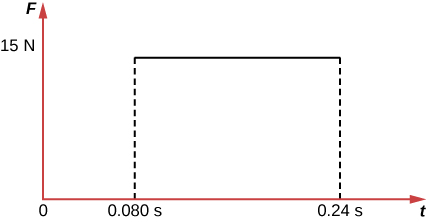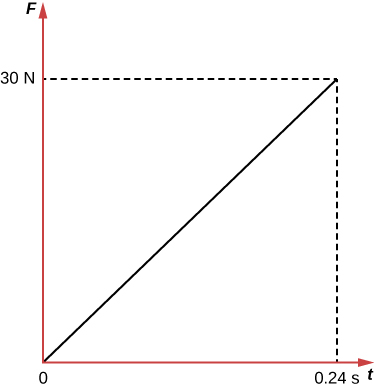# 8.2 Impulse  (Page 2/8)

 Page 2 / 8

## Making connections: illustrations of force exertedThis is a graph showing the force exerted by a fixed barrier on a block versus time.

A 1.2-kg block slides across a horizontal, frictionless surface with a constant speed of 3.0 m/s before striking a fixed barrier and coming to a stop. In [link] , the force exerted by the barrier is assumed to be a constant 15 N during the 0.24-s collision. The impulse can be calculated using the area under the curve.

Note that the initial momentum of the block is:

We are assuming that the initial velocity is −3.0 m/s. We have established that the force exerted by the barrier is in the positive direction, so the initial velocity of the block must be in the negative direction. Since the final momentum of the block is zero, the impulse is equal to the change in momentum of the block.

Suppose that, instead of striking a fixed barrier, the block is instead stopped by a spring.Consider the force exerted by the spring over the time interval from the beginning of the collision until the block comes to rest.This is a graph showing the force exerted by a spring on a block versus time.

In this case, the impulse can be calculated again using the area under the curve (the area of a triangle):

Again, this is equal to the difference between the initial and final momentum of the block, so the impulse is equal to the change in momentum.

## Calculating magnitudes of impulses: two billiard balls striking a rigid wall

Two identical billiard balls strike a rigid wall with the same speed, and are reflected without any change of speed. The first ball strikes perpendicular to the wall. The second ball strikes the wall at an angle of $\text{30º}$ from the perpendicular, and bounces off at an angle of $\text{30º}$ from perpendicular to the wall.

(a) Determine the direction of the force on the wall due to each ball.

(b) Calculate the ratio of the magnitudes of impulses on the two balls by the wall.

Strategy for (a)

In order to determine the force on the wall, consider the force on the ball due to the wall using Newton’s second law and then apply Newton’s third law to determine the direction. Assume the $x$ -axis to be normal to the wall and to be positive in the initial direction of motion. Choose the $y$ -axis to be along the wall in the plane of the second ball’s motion. The momentum direction and the velocity direction are the same.

Solution for (a)

The first ball bounces directly into the wall and exerts a force on it in the $+x$ direction. Therefore the wall exerts a force on the ball in the $-x$ direction. The second ball continues with the same momentum component in the $y$ direction, but reverses its $x$ -component of momentum, as seen by sketching a diagram of the angles involved and keeping in mind the proportionality between velocity and momentum.

These changes mean the change in momentum for both balls is in the $-x$ direction, so the force of the wall on each ball is along the $-x$ direction.

Why does earth exert only a tiny downward pull?
hello
Islam
Why is light bright?
an 8.0 capacitor is connected by to the terminals of 60Hz whoes rms voltage is 150v. a.find the capacity reactance and rms to the circuit
thanks so much. i undersooth well
what is physics
is the study of matter in relation to energy
Kintu
a submersible pump is dropped a borehole and hits the level of water at the bottom of the borehole 5 seconds later.determine the level of water in the borehole
what is power?
power P = Work done per second W/ t. It means the more power, the stronger machine
Sphere
e.g. heart Uses 2 W per beat.
Rohit
A spherica, concave shaving mirror has a radius of curvature of 32 cm .what is the magnification of a persons face. when it is 12cm to the left of the vertex of the mirror
did you solve?
Shii
1.75cm
Ridwan
my name is Abu m.konnek I am a student of a electrical engineer and I want you to help me
Abu
the magnification k = f/(f-d) with focus f = R/2 =16 cm; d =12 cm k = 16/4 =4
Sphere
what do we call velocity
Kings
A weather vane is some sort of directional arrow parallel to the ground that may rotate freely in a horizontal plane. A typical weather vane has a large cross-sectional area perpendicular to the direction the arrow is pointing, like a “One Way” street sign. The purpose of the weather vane is to indicate the direction of the wind. As wind blows pa
hi
Godfred
Godfred
If a prism is fully imersed in water then the ray of light will normally dispersed or their is any difference?
the same behavior thru the prism out or in water bud abbot
Ju
If this will experimented with a hollow(vaccum) prism in water then what will be result ?
Anurag
What was the previous far point of a patient who had laser correction that reduced the power of her eye by 7.00 D, producing a normal distant vision power of 50.0 D for her?
What is the far point of a person whose eyes have a relaxed power of 50.5 D?
Jaydie
What is the far point of a person whose eyes have a relaxed power of 50.5 D?
Jaydie
A young woman with normal distant vision has a 10.0% ability to accommodate (that is, increase) the power of her eyes. What is the closest object she can see clearly?
Jaydie
29/20 ? maybes
Ju
In what ways does physics affect the society both positively or negatively
how can I read physics...am finding it difficult to understand...pls help
try to read several books on phy don't just rely one. some authors explain better than other.
Ju
And don't forget to check out YouTube videos on the subject. Videos offer a different visual way to learn easier.
Ju
hope that helps
Ju
I have a exam on 12 february
what is velocity
Jiti
the speed of something in a given direction.
JuByByByBy Subramanian DivyaByBy